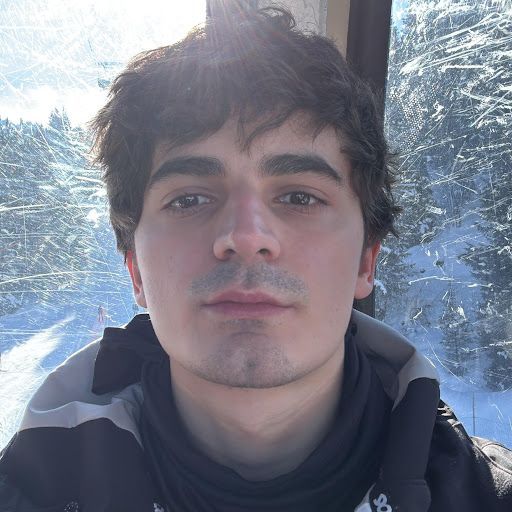C. Cristi

Bucharest, Romania

Freshman student of Automatics and Computer Science with great ambition and teamwork skills gained in volunteering.

Hard working, highly passionate computer science student, problem solver enthusiast, interested in data structures, algorithms. Enjoying basketball, art, photography, calisthenics and playing video games.

Top Questions

Calculate an abstract algebra “sum” $\frac 12 * \frac13 *…*\frac 1{1000}$.

asked May 18 '18 at 12:01

Find $B=A^2+A$ knowing that $A^3=\begin{bmatrix}4&3\\-3&-2\end{bmatrix}$

asked Jul 2 '18 at 6:09

Compute $\lim_{n\rightarrow\infty}\frac{n^n}{(n!)^2}$

asked Apr 8 '18 at 8:57

Number of elements in the set $A=\{x\in\mathbb{R}|f(x)=1\}$.

asked Jun 16 '18 at 16:31

Find $\operatorname{Tr}(A^{2018})$ if $\det(A^2-2018I_2)=0$

asked Jul 8 '18 at 17:44